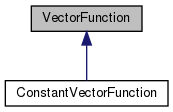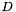Chombo + EB + MF  3.2
VectorFunction Class Referenceabstract

#include <VectorFunction.H>

Inheritance diagram for VectorFunction:[legend]

Public Member Functions

VectorFunction (bool a_homogeneous, bool a_constant)

virtual ~VectorFunction ()
Destructor. More...

virtual RealVect operator() (const RealVect &a_x, Real a_t) const =0

virtual RealVect derivative (const IntVect &a_order, const RealVect &a_x, Real a_t) const

virtual bool hasDerivative (const IntVect &a_order) const

RealVect operator() (const RealVect &a_x) const

RealVect derivative (const IntVect &a_order, const RealVect &a_x) const

bool isHomogeneous () const
Returns true if this function is homogeneous, false otherwise. More...

bool isConstant () const
Returns true if this function is constant, false otherwise. More...

Protected Attributes

bool m_isHomogeneous

bool m_isConstant

Private Member Functions

VectorFunction ()

VectorFunction (const VectorFunction &)

VectorFunctionoperator= (const VectorFunction &)

Detailed Description

This base class represents a vector function.

Constructor & Destructor Documentation

 VectorFunction::VectorFunction ( bool a_homogeneous, bool a_constant )

Base class constructor. Must be called by subclasses.

Parameters
 a_homogeneous This flag indicates whether the vector function is constant in space. a_constant This flag indicates whether the vector function is constant in time.
 virtual VectorFunction::~VectorFunction ( )
virtual

Destructor.

 VectorFunction::VectorFunction ( )
private
 VectorFunction::VectorFunction ( const VectorFunction & )
private

Member Function Documentation

 virtual RealVect VectorFunction::operator() ( const RealVect & a_x, Real a_t ) const
pure virtual

Override this method to evaluate this function at the given point in space and time.

Parameters
 a_x A point in-dimensional space. a_t The time at which the function is evaluated.

Implemented in ConstantVectorFunction.

Referenced by operator()().

 virtual RealVect VectorFunction::derivative ( const IntVect & a_order, const RealVect & a_x, Real a_t ) const
virtual

Override this method to evaluate the given partial derivative of the function at the given point in space and time.

Parameters
 a_order A multi-index identifying the order(s) of the partial derivative of the function to be evaluated. a_x A point in-dimensional space. a_t The time at which the derivative is to be evaluated.

Reimplemented in ConstantVectorFunction.

Referenced by derivative().

 virtual bool VectorFunction::hasDerivative ( const IntVect & a_order ) const
virtual

Override this method to return true if the derivative of the requested order exists and is available, false if it is not. This must be implemented in a way that is consistent with the derivative method.

Parameters
 a_order A multi-index identifying the order(s) of the desired partial derivative of the function.
 RealVect VectorFunction::operator() ( const RealVect & a_x ) const
inline

This evaluates the function at time 0.

Parameters
 a_x A point in-dimensional space.

References operator()().

 RealVect VectorFunction::derivative ( const IntVect & a_order, const RealVect & a_x ) const
inline

This evaluates the given partial derivative of the function at time 0.

Parameters
 a_order A multi-index identifying the order(s) of the partial derivative of the function to be evaluated. a_x A point in-dimensional space.

References derivative().

 bool VectorFunction::isHomogeneous ( ) const
inline

Returns true if this function is homogeneous, false otherwise.

References m_isHomogeneous.

 bool VectorFunction::isConstant ( ) const
inline

Returns true if this function is constant, false otherwise.

References m_isConstant.

 VectorFunction& VectorFunction::operator= ( const VectorFunction & )
private

Member Data Documentation

 bool VectorFunction::m_isHomogeneous
protected

Referenced by isHomogeneous().

 bool VectorFunction::m_isConstant
protected

Referenced by isConstant().

The documentation for this class was generated from the following file: